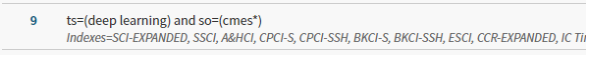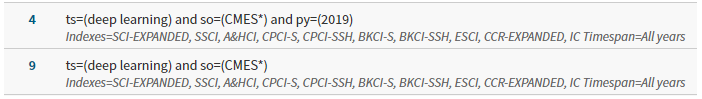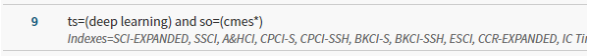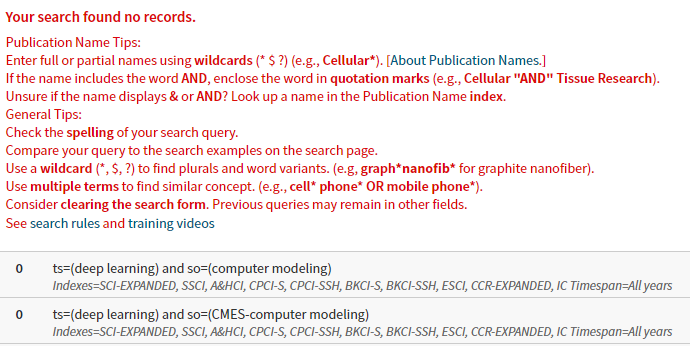Web of Science Search Methods for CMES

1 Recommended search method

To search certain topic, e.g., deep learning, in the CMES (Computer Modeling in Engineering & Sciences) in the Web of Science database, a most efficient method is to specify the source (journal title) as “cmes*”, i.e., all in lowercase followed by the wildcard “*” (star), as shown in the search example below:

 ts=(deep learning) and so=(cmes*)2 Correct search methods

The correct and simplest search method is to declare the source (journal title) as “CMES*”. The wildcard star “*” at the end of “CMES” is important for the search to be successful:

ts=(deep learning) and so=(CMES*) and py=(2019)

ts=(deep learning) and so=(CMES*)The journal title can be in lowercase, e.g.,

 ts=(deep learning) and so=(cmes*)It is possible to cite the source with the full journal name, with or without hyphen, but that is long and mistyping is easy:

ts=(deep learning) and so=(cmes computer modeling in engineering & sciences)

ts=(deep learning) and so=(cmes-computer modeling in engineering & sciences)3 Incorrect search methods

ts=(deep learning) and so=(computer modeling)

ts=(deep learning) and so=(CMES-computer modeling)The following search also does not work:

ts=(deep learning) and so=(*computer modeling in*)

ts=(deep learning) and so=(computer modeling in*)